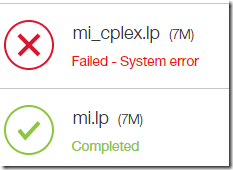## Tuesday, April 21, 2015

### Scary numbers

In http://lists.gnu.org/archive/html/help-glpk/2015-04/msg00031.html we see GLPK failing with some scary looking messages:

With glpk 4.52 and with the attached input, I get the following failure/error:

------------------------------------------------------------------------------
\$ glpsol --lp mi.cplex

Warning: numerical instability (primal simplex, phase I)
2979: obj =   1.092652474e+04  infeas =  5.265e+09 (0)
Warning: numerical instability (primal simplex, phase I)
2996: obj =   1.092637382e+04  infeas =  5.260e+09 (0)
3000: obj =   1.092644011e+04  infeas =  5.235e+09 (0)
Warning: numerical instability (primal simplex, phase I)
3051: obj =   1.077725595e+04  infeas =  5.201e+09 (0)
Warning: numerical instability (primal simplex, phase I)
3059: obj =   1.074805814e+04  infeas =  5.185e+09 (0)
Warning: numerical instability (primal simplex, phase I)
3122: obj =   1.998434476e+03  infeas =  3.955e+05 (0)
Warning: numerical instability (primal simplex, phase I)
3159: obj =   1.998431624e+03  infeas =  3.944e+05 (0)
Error: unable to factorize the basis matrix (1)
Sorry, basis recovery procedure not implemented yet
glp_intopt: cannot solve LP relaxation
Time used:   1.7 secs
Memory used: 10.8 Mb (11322915 bytes)
--------------------------------------------------------------

Any insights/workarounds will be appreciated.

Even more scary is the following part of the log:

Scaling...
A: min|aij| =  1.000e+00  max|aij| =  2.306e+18  ratio =  2.306e+18
GM: min|aij| =  1.213e-04  max|aij| =  8.246e+03  ratio =  6.800e+07
EQ: min|aij| =  1.480e-08  max|aij| =  1.000e+00  ratio =  6.755e+07
2N: min|aij| =  1.490e-08  max|aij| =  1.500e+00  ratio =  1.007e+08
Commercial solvers typically do much better on these kind of poorly scaled models. As the attached problem file was called mi.cplex, I tried Cplex’s DropSolve on-line tool on this problem. It solves it quickly:

Changed parameter CPX_PARAM_THREADS from 0 to 12
Param[1,067] = 12
Param[1,130] = UTF-8
Param[1,132] = -1
Tried aggregator 1 time.
MIP Presolve eliminated 535 rows and 0 columns.
MIP Presolve modified 38 coefficients.
Reduced MIP has 4312 rows, 345 columns, and 48488 nonzeros.
Reduced MIP has 152 binaries, 193 generals, 0 SOSs, and 0 indicators.
Presolve time = 0.02 sec. (16.48 ticks)
Found incumbent of value 1.2193885e+12 after 0.05 sec. (30.35 ticks)
Probing time = 0.11 sec. (6.03 ticks)
Tried aggregator 1 time.
Reduced MIP has 4312 rows, 345 columns, and 48488 nonzeros.
Reduced MIP has 152 binaries, 193 generals, 0 SOSs, and 0 indicators.
Presolve time = 0.01 sec. (11.80 ticks)
Probing time = 0.11 sec. (5.81 ticks)
Clique table members: 744.
MIP emphasis: balance optimality and feasibility.
MIP search method: dynamic search.
Parallel mode: deterministic, using up to 12 threads.
Root relaxation solution time = 0.08 sec. (17.25 ticks)

Nodes                                         Cuts/
Node  Left     Objective  IInf  Best Integer    Best Bound    ItCnt     Gap

*     0+    0                       1.21939e+12     -228.0000           100.00%
*     0+    0                       1.21939e+12     -228.0000           100.00%
*     0+    0                       1.21939e+12     -228.0000           100.00%
0     0        0.0000    76   1.21939e+12        0.0000      190  100.00%
0     0       22.2000   114   1.21939e+12     Fract: 76      663  100.00%
*     0+    0                       6.45608e+11       22.2000           100.00%
0     2       22.2000   114   6.45608e+11       22.2000      663  100.00%
Elapsed time = 1.08 sec. (582.03 ticks, tree = 0.00 MB, solutions = 4)
*     3+    3                       137054.0000       23.0197            99.98%
3     5      820.0414   170   137054.0000       23.0197     2837   99.98%
13    15      963.0516   223   137054.0000      822.1327     5049   99.40%
*   156   119      integral     0     4281.0000      822.1327    12517   80.80%
160    65     4266.0500   109     4281.0000      858.1613    12976   79.95%
*   197    31      integral     0     4278.0000      858.1613    13771   79.94%
*   207    21      integral     0     4275.0000      858.1613    13951   79.93%

Gomory fractional cuts applied:  76

Root node processing (before b&c):
Real time             =    1.06 sec. (578.93 ticks)
Parallel b&c, 12 threads:
Real time             =    1.62 sec. (1062.86 ticks)
Sync time (average)   =    1.06 sec.
Wait time (average)   =    1.10 sec.
------------
Total (root+branch&cut) =    2.67 sec. (1641.79 ticks)
Incumbent solution:
MILP objective                                 4.2750000000e+03
MILP solution norm |x| (Total, Max)            1.36000e+02  6.00000e+00
MILP solution error (Ax=b) (Total, Max)        2.02749e-12  1.13687e-13
MILP x bound error (Total, Max)                3.15081e-13  1.05027e-13
MILP x integrality error (Total, Max)          1.22169e-12  2.08722e-13
MILP slack bound error (Total, Max)            7.46694e-11  1.98952e-12
integer optimal solution (101)

I would certainly double check the solution.

Note: The first run on DropSolve failed. I renamed the file (not sure if the filename contributed to the problem), and tried again. Now it worked: